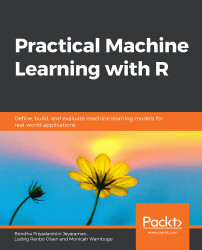•#### Practical Machine Learning with R#### Overview of this book

With huge amounts of data being generated every moment, businesses need applications that apply complex mathematical calculations to data repeatedly and at speed. With machine learning techniques and R, you can easily develop these kinds of applications in an efficient way. Practical Machine Learning with R begins by helping you grasp the basics of machine learning methods, while also highlighting how and why they work. You will understand how to get these algorithms to work in practice, rather than focusing on mathematical derivations. As you progress from one chapter to another, you will gain hands-on experience of building a machine learning solution in R. Next, using R packages such as rpart, random forest, and multiple imputation by chained equations (MICE), you will learn to implement algorithms including neural net classifier, decision trees, and linear and non-linear regression. As you progress through the book, you’ll delve into various machine learning techniques for both supervised and unsupervised learning approaches. In addition to this, you’ll gain insights into partitioning the datasets and mechanisms to evaluate the results from each model and be able to compare them. By the end of this book, you will have gained expertise in solving your business problems, starting by forming a good problem statement, selecting the most appropriate model to solve your problem, and then ensuring that you do not overtrain it.Free Chapter
An Introduction to Machine LearningData Cleaning and Pre-processingFeature EngineeringIntroduction to neuralnet and Evaluation MethodsLinear and Logistic Regression ModelsUnsupervised LearningAppendix## DIANA

DIANA stands for DIvisive ANAlysis clustering. It is a hierarchical clustering technique that starts with one cluster and subsequently divides the clusters until each cluster is just a single element. At each step, we select the cluster with the most dissimilar elements. The most dissimilar element within that cluster is then used as a starting point in the creation of a new cluster, consequently splitting the original cluster into two. Divisive coefficient is a unit that measures the amount of clustering structures found.

#### Note

For more information on DIANA, please refer to the work of Kaufman and Rousseeuw (1990), Finding groups in data. Rousseeuw, Peter J., and L. Kaufman. "Finding groups in data." Hoboken: Wiley Online Library (1990).

In the next exercise, we will be covering the concepts of hierarchical clustering using DIANA.

### Exercise 61: Implement Hierarchical Clustering Using DIANA

In this exercise, we will perform hierarchal clustering using DIANA. We will be using the...